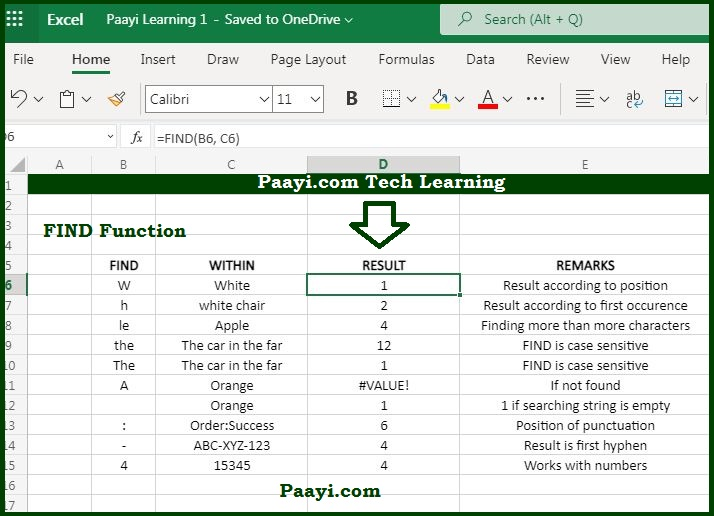# Learn How to Use Microsoft Excel FIND Function

Written by | 0 Comments | 671 Views

In this article, you will learn how to use the Microsoft Excel FIND function and its prime function in Microsoft Excel. You will also get to know the Microsoft Excel FIND function return value and syntax with the help of some examples.

## Microsoft Excel FIND Function

The main purpose of the Microsoft Excel FIND function to get the location of a text in a string. That means with the help of the FIND function, you can able to return the position as a number of one text string within another as a return value. In case the text is not found, the FIND function will return a #VALUE error.

### FIND Function Return Value

The return value will be a number representing the location of the find-text.

### FIND Function Syntax

= FIND(find-text, within-text, [start-num])

Where the arguments:

• find-text: It is the text to find out.
• within-text: It is text in which we want to search.
• start-num: (Optional) It is the starting position in the text to search, Optional defaults to 1.

## How to Use Microsoft Excel FIND Function?As we know, the FIND function returns the position (as a number) of one text string inside another. In case there is more than one occurrence of the text string, the position (as a number) of one text string inside another function the position of the first occurrence.

The FIND function does not support the wildcards, and it is always case-sensitive. You can use the SEARCH function to find the position of the text without being case-sensitive and have wildcard support. In case the text is not found, the FIND function will return the #VALUE error. Besides that, if find-text is empty, the return value will be 1.

Let us go through some of the examples of the FIND function as given below:

Example 1: = FIND("A", "BANANA") - This will return the value 1.

Example 2: = FIND("The", "The cat in the hat") - This will return value 12.